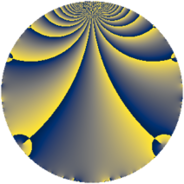# Properties

 Label 385.2.aLevel $385$ Weight $2$ Character orbit 385.a Rep. character $\chi_{385}(1,\cdot)$ Character field $\Q$ Dimension $19$ Newform subspaces $8$ Sturm bound $96$ Trace bound $3$

# Related objects

## Defining parameters

 Level: $$N$$ $$=$$ $$385 = 5 \cdot 7 \cdot 11$$ Weight: $$k$$ $$=$$ $$2$$ Character orbit: $$[\chi]$$ $$=$$ 385.a (trivial) Character field: $$\Q$$ Newform subspaces: $$8$$ Sturm bound: $$96$$ Trace bound: $$3$$ Distinguishing $$T_p$$: $$2$$, $$3$$

## Dimensions

The following table gives the dimensions of various subspaces of $$M_{2}(\Gamma_0(385))$$.

Total New Old
Modular forms 52 19 33
Cusp forms 45 19 26
Eisenstein series 7 0 7

The following table gives the dimensions of the cuspidal new subspaces with specified eigenvalues for the Atkin-Lehner operators and the Fricke involution.

$$5$$$$7$$$$11$$FrickeDim.
$$+$$$$+$$$$+$$$$+$$$$3$$
$$+$$$$+$$$$-$$$$-$$$$2$$
$$+$$$$-$$$$+$$$$-$$$$2$$
$$+$$$$-$$$$-$$$$+$$$$3$$
$$-$$$$+$$$$+$$$$-$$$$4$$
$$-$$$$+$$$$-$$$$+$$$$1$$
$$-$$$$-$$$$+$$$$+$$$$1$$
$$-$$$$-$$$$-$$$$-$$$$3$$
Plus space$$+$$$$8$$
Minus space$$-$$$$11$$

## Trace form

 $$19q - 3q^{2} - 4q^{3} + 21q^{4} - q^{5} + 20q^{6} - q^{7} + 9q^{8} + 7q^{9} + O(q^{10})$$ $$19q - 3q^{2} - 4q^{3} + 21q^{4} - q^{5} + 20q^{6} - q^{7} + 9q^{8} + 7q^{9} + 5q^{10} - q^{11} - 4q^{12} - 14q^{13} - 3q^{14} + 4q^{15} + 13q^{16} - 10q^{17} - 15q^{18} + 4q^{19} - 7q^{20} - 4q^{21} + q^{22} + 16q^{23} + 12q^{24} + 19q^{25} - 10q^{26} + 8q^{27} - 7q^{28} - 22q^{29} - 12q^{30} - 8q^{31} - 15q^{32} - 4q^{33} - 14q^{34} - q^{35} - 15q^{36} - 22q^{37} - 36q^{38} + 8q^{39} + 33q^{40} - 2q^{41} - 4q^{42} - 20q^{43} - 7q^{44} + 3q^{45} - 24q^{47} - 52q^{48} + 19q^{49} - 3q^{50} - 8q^{51} - 26q^{52} + 10q^{53} + 32q^{54} - q^{55} - 15q^{56} - 16q^{57} + 14q^{58} + 36q^{59} - 4q^{60} - 14q^{61} + 56q^{62} + 3q^{63} + 93q^{64} - 6q^{65} - 12q^{66} - 4q^{67} - 46q^{68} + 16q^{69} + q^{70} + 8q^{71} - 19q^{72} - 34q^{73} + 46q^{74} - 4q^{75} - 4q^{76} + 7q^{77} + 8q^{78} + q^{80} - 21q^{81} + 26q^{82} + 4q^{83} + 28q^{84} + 14q^{85} - 52q^{86} + 8q^{87} - 3q^{88} - 2q^{89} - 7q^{90} + 2q^{91} + 40q^{92} + 48q^{93} + 56q^{94} + 4q^{95} - 76q^{96} - 18q^{97} - 3q^{98} - 13q^{99} + O(q^{100})$$

## Decomposition of $$S_{2}^{\mathrm{new}}(\Gamma_0(385))$$ into newform subspaces

Label Dim. $$A$$ Field CM Traces A-L signs $q$-expansion
$$a_2$$ $$a_3$$ $$a_5$$ $$a_7$$ 5 7 11
385.2.a.a $$1$$ $$3.074$$ $$\Q$$ None $$-1$$ $$-2$$ $$1$$ $$1$$ $$-$$ $$-$$ $$+$$ $$q-q^{2}-2q^{3}-q^{4}+q^{5}+2q^{6}+q^{7}+\cdots$$
385.2.a.b $$1$$ $$3.074$$ $$\Q$$ None $$-1$$ $$0$$ $$1$$ $$-1$$ $$-$$ $$+$$ $$-$$ $$q-q^{2}-q^{4}+q^{5}-q^{7}+3q^{8}-3q^{9}+\cdots$$
385.2.a.c $$2$$ $$3.074$$ $$\Q(\sqrt{3})$$ None $$0$$ $$2$$ $$-2$$ $$2$$ $$+$$ $$-$$ $$+$$ $$q+\beta q^{2}+(1+\beta )q^{3}+q^{4}-q^{5}+(3+\beta )q^{6}+\cdots$$
385.2.a.d $$2$$ $$3.074$$ $$\Q(\sqrt{2})$$ None $$2$$ $$0$$ $$-2$$ $$-2$$ $$+$$ $$+$$ $$-$$ $$q+(1+\beta )q^{2}+\beta q^{3}+(1+2\beta )q^{4}-q^{5}+\cdots$$
385.2.a.e $$3$$ $$3.074$$ 3.3.148.1 None $$-3$$ $$-4$$ $$-3$$ $$3$$ $$+$$ $$-$$ $$-$$ $$q+(-1-\beta _{2})q^{2}+(-1-\beta _{1})q^{3}+(2+\cdots)q^{4}+\cdots$$
385.2.a.f $$3$$ $$3.074$$ 3.3.148.1 None $$-3$$ $$-2$$ $$-3$$ $$-3$$ $$+$$ $$+$$ $$+$$ $$q+(-1-\beta _{2})q^{2}+(-1+\beta _{1})q^{3}+(2+\cdots)q^{4}+\cdots$$
385.2.a.g $$3$$ $$3.074$$ 3.3.148.1 None $$1$$ $$0$$ $$3$$ $$3$$ $$-$$ $$-$$ $$-$$ $$q+\beta _{1}q^{2}+\beta _{2}q^{3}+(\beta _{1}+\beta _{2})q^{4}+q^{5}+\cdots$$
385.2.a.h $$4$$ $$3.074$$ 4.4.11348.1 None $$2$$ $$2$$ $$4$$ $$-4$$ $$-$$ $$+$$ $$+$$ $$q+(1+\beta _{2})q^{2}+(1+\beta _{3})q^{3}+(2+\beta _{2}+\cdots)q^{4}+\cdots$$

## Decomposition of $$S_{2}^{\mathrm{old}}(\Gamma_0(385))$$ into lower level spaces

$$S_{2}^{\mathrm{old}}(\Gamma_0(385)) \cong$$ $$S_{2}^{\mathrm{new}}(\Gamma_0(11))$$$$^{\oplus 4}$$$$\oplus$$$$S_{2}^{\mathrm{new}}(\Gamma_0(35))$$$$^{\oplus 2}$$$$\oplus$$$$S_{2}^{\mathrm{new}}(\Gamma_0(55))$$$$^{\oplus 2}$$$$\oplus$$$$S_{2}^{\mathrm{new}}(\Gamma_0(77))$$$$^{\oplus 2}$$